Control of a Batch Process Practical Application of the Polynomial Toolbox in the film processing industry (the Kodak Corp.)

1) Signals, Systems and Control Department
Faculty of Mathematical Sciences
University of Twente
The Netherlands

2) Kodak Corporation

 Introduction Design Targets Theory Process and Disturbance Models Algorithm Application to the Batch Process Computation Spectral Facrtorization Two-sided Equation The Controller Response to Disturbances Impulse and Step Responses Assesments

Introduction
Many photographic films and papers are manufactured in a batch-like mode. In this mode batches of sensitized material are made up and then coated onto a base. To guarantee that the photographic properties are kept within limits, strips of product are regularly sent to testing for assessment. If the product is drifting off aim then it is possible to add dye or change the laydown to move the product back on target.

However, there frequently are more outputs than ”control knobs” to use for adjustment, and the inputs frequently affect many outputs simultaneously. Testing delay, more outputs than inputs, the desire for a first-order return rather than a step return to target for some products, and stochastic disturbances make this an interesting control problem.

Below is the transfer function plus disturbance model for a process like the one described above.Here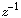is the delay operator and the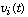are disturbance signals.

The three outputs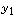,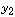andsuccessively are the deviations from aim of photographic speed, contrast, and upper density point. The two inputs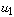and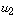are dye and laydown changes from preset starting values, and the delay of three accounts for the zero order hold and testing delay.

The three disturbance models are first order integrated moving average, first order integrated autoregressive, and first order integrated moving average, respectively.

Design Targets
We look for a feedback controller that

• reduces the effect of random disturbances, and
• eliminates the effect of long term drifts in the disturbances

Theory
A useful control design approach is the Internal Model Control (IMC) approach discussed in MacGregor and Harris (1987). Fig. 1 shows the arrangement.

The control law H for this problem follows by the minimization of a mean square error criterion of the form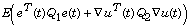E denotes the expectation, e(t) is the deviation of the output y(t) from its set point, and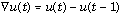is the incremental control action.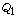and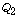are weighting matrices.Fig. 1. Internal Model Control

Process & Disturbance Models
The control is computed by a polynomial algorithm. To this end, the plant-disturbance model is rendered aswith D(t) the effect of the disturbances on the output y. The process model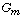is described by the right polynomial matrix fraction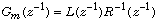with L and R polynomial matrices in the delay operator. The disturbance model is taken as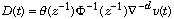with v a column vector of white noise inputs. The quantitiesand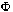are polynomial matrices in the delay operator, withdiagonal, andAlgorithm
The first step of the algorithm is to find the approximate inverse model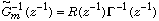for the plant. The polynomial matrixfollows by the spectral factorization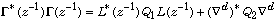The superscript * denotes the adjoint, that is,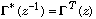The spectral factorneeds to have all its roots outside the unit circle.

The controller H may now be expressed as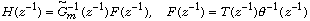where the polynomial matrix T, together with the polynomial matrix P, is the solution of the two-sided equation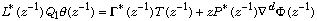Application to the Batch Process Problem
To apply the algorithm to the batch control problem we need to obtain the process and disturbance models in the required form. Inspection of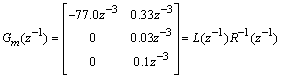reveals that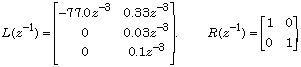Furthermore, writing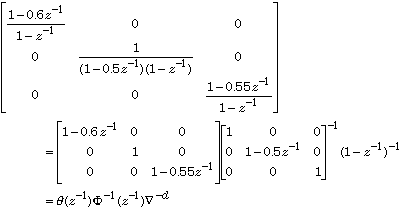we see that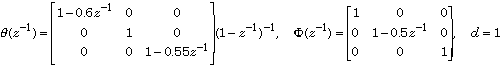The weighting matrices, finally, are selected as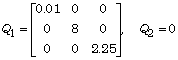Computation
The first step of the computation is to define the input data. Thus, the first few lines of the script demoB.m are

% demoB
% Script for the demo "Control of a batch process"

% Define the data
L = [-77*z^-3 0.33*z^-3; 0 0.03*z^-3; 0 0.1*z^-3];
R = eye(2,2);
theta = diag([1-0.6*z^-1 1 1-0.55*z^-1]);
Phi = diag([1 1-0.5*z^-1 1]);
d = 1;
Q1 = diag([0.01 8 2.25]);
Nabla = (1-z^-1);

Spectral Factorization
The first computational step is to determine the polynomial matrix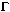by spectral factorization:

% Spectral factorization
Gamma = spf(L'*Q1*L);

The result is

Gamma

Constant polynomial matrix: 2-by-2
Gamma =
7.7 -0.033
0.00074 0.17

Solution of the Two-sided Equation
The next computational step is to solve the two-sided matrix equationfor the matrices T and P. For this we use the routine axybc. This solves the two-sided polynomial matrix equationTo turn into a polynomial matrix equation we need to multiply it by a suitable power n ofso that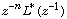and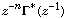both are polynomial and also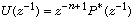is a polynomial matrix.

Choosing n = 3 we have

% Solution of the two-sided equation
n = 3;
A = z^-n*Gamma';
B = Nabla^d*Phi;
C = L'*Q1*theta;
[T,U] = axybc(A,B,C);

T turns out to be 2×3 matrix of degree 1, as predicted by the theory (MacGregor and Harris, 1987):

T =

-0.04 -0.00025 + 0.00012z^-1 -5.6e-005
-3.8e-006 2.6 - 1.2z^-1 0.59

The Controller
The controller transfer matrix is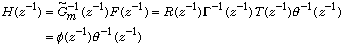where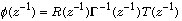is polynomial becauseis a constant matrix. We compute the controller as

% Compute the controller H = phi/theta
phi = R/Gamma*T;

The result is

phi =

-0.0052 0.065 - 0.03z^-1 0.015
-1.3e-016 15 - 7.1z^-1 3.4

Response to Disturbances
We study the effect of disturbances D(t) at the plant output such that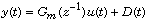The closed-loop response to the disturbances D(t) follows from the sensitivity matrix S: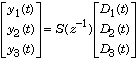It is not difficult to find from the block diagram of Fig. 1 that if the plant model exactly matches the plant then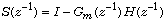It follows that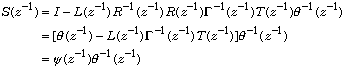where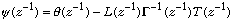% Compute the sensitivity matrix S = psi/theta
psi = theta-L/Gamma*T;

Disturbance Impulse & Step Responses
The Control Toolbox and even more Simulink are very well equipped to compute, plot and manipulate time responses. We stay within the confines of the Polynomial Toolbox, however, and use long polynomial division to compute the impulse and step responses to disturbances (see the demo "Computing the covariance function of an ARMA process".)

% Compute and plot the disturbance impulse response matrix r
% Apply long division to psi/theta
n = 10;
[q,r] = longrdiv(psi,theta,n);

% Plot the data
figure; clf
k = length(r);

for i = 1:k
for j = 1:k
subplot(k,k,(i-1)*k+j)
plot(0:n,r{:}(i,j))
grid on; axis([0 n -1.5 1.5])
end
end

Matlab produces the plot of Fig. 2 for the impulse response matrix. The step responses may be computed similarly and are shown in Fig. 3.

% Compute and plot the disturbance step response matrix s
% Apply long division to (psi/theta)*1/(1-z^-1)
n = 10;
[q,s] = longrdiv(psi,theta*(1-z^-1),n);

% Plot
figure; clf
k = length(s);
for i = 1:k
for j = 1:k
subplot(k,k,(i-1)*k+j)
plot(0:n,s{:}(i,j))
grid on; axis([0 n -1.5 1.5])
end
end

•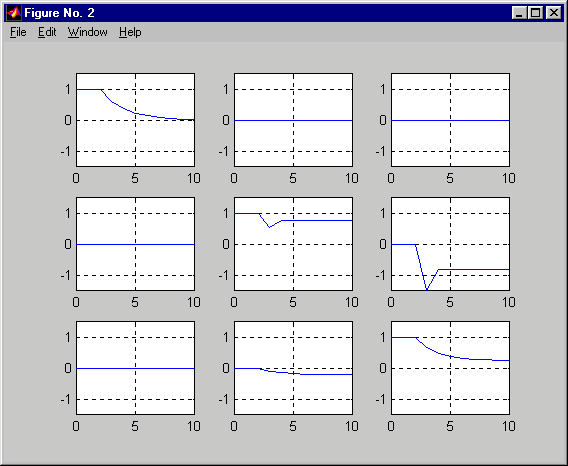• Fig. 2. Disturbance impulse response matrixFig. 3. Disturbance step response matrix

Assesment
Inspection of the step response matrix shows this:

• The (1,2) and (1,3) entries of the step response matrix are identical to zero. This means that the first output is insensitive to the second and third components of the disturbances.
• Likewise, the (2,1) and (3,1) entries are zero. This means that the second and third outputs are insensitive to the first component of the disturbance.
• All nonzero entries show a dead time of three time steps. This is a consequence of the dead time of the process.
• The step response in the (1,1) entry eventually goes to zero. This means that constant disturbances are suppressed in this channel.
• The step responses in the remaining zero entries do not approach zero, which means that constant disturbances in the second and third channel are not suppressed. The reason for this is that there are three components to the disturbance but only two control inputs so that a full degree of freedom is lacking. The resulting loss in performance is divided between the second and third channels. The balance of this division may be shifted by adjusting the entries of the weighting matrix